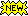[close ]
[close ]
[ close ]
[ close ]
[ close ]
[ close ]
[ close ]

Labeling this number of sequences as 'positive' was found by AME to be optimal (or your primary sequence file contains this number of sequences and you also provided a control sequence file). The sequences were chosen as described above under "Labeling positives".

[ close ]

Labeling this number of sequences as 'negative' was found by AME to be optimal (or you provided a control sequence file containing this number of sequences). The sequences are those remaining after the 'positive' sequences were chosen as described above under "Labeling positives".

[ close ]

The number (percentage) of sequences labeled as 'positive' (Pos) and classified as 'positive' (TP),
%TP = 100 * TP/Pos.
Labeling of sequences as 'positive' is described in the help bubble for "Pos". Classification is described above under "Classifying positives".

[ close ]

The number (percentage) of sequences labeled as 'negative' (Neg) but classified as 'positive' (FP),
%FP = 100 * FP/Neg.
Labeling of sequences as 'negative' is described in the help bubble for "Neg". Classification is described above under "Classifying positives".

[ close ]

The (optimal) score threshold for labeling sequences as 'positive' determined by AME. (See the note about "Labeling positives" in the section above.)

[ close ]

The (optimal) score threshold for classifying sequences as 'positive' determined by AME (or provided by you using `--hit-odds-fraction` or `--fasta-threshold`).
By default, the true positive and false positive sequences are those with sequence motif scores ≥ the value shown.
If you specified `--poslist pwm`, then the true positive and false positive sequences are those with FASTA scores ≤ the value shown.

[ close ]
[ close ]
[ close ]

The value of Pearson's correlation coefficient (ρ). It varies between -1 and +1. High values of ρ indicate strong correlation between the sequence motif scores and the sequence FASTA scores. Note that positive ρ means that sequence motif scores go up when sequence FASTA scores go down. The p-value of this method is the probability that ρ is at least as large as the observed value assuming there is no relation between the scores.

[ close ]

The value of Spearman's rank correlation coefficient (ρ). It varies between -1 and +1. High values of ρ indicate strong correlation between the sequence motif score ranks and the sequence FASTA score ranks. Note that positive ρ means that sequence motif scores go up when sequence FASTA scores go down. The p-value of this method is the probability that ρ is at least as large as the observed value assuming there is no relation between the scores.

[ close ]

The value of the mean squared error of the linear regression of X vs Y.

[ close ]

The slope of the regression line: Y = mX + b.

[ close ]

The Y-intercept of the regression line: Y = mX + b.

[ close ]

For further information on how to interpret these results please access https://meme-suite.org/meme/doc/ame-output-format.html.
To get a copy of the MEME software please access https://meme-suite.org.

If you use AME in your research, please cite the following paper:
Robert McLeay and Timothy L. Bailey, "Motif Enrichment Analysis: A unified framework and method evaluation", BMC Bioinformatics, 11:165, 2010, doi:10.1186/1471-2105-11-165. [full text]

Enriched Motifs   |   Input Files   |   Program information   |   Results in TSV Format## Enriched Motifs

Database
ID
Alt ID
p-value
E-value
Pos Thresh
Pos
Neg
TP Thresh
TP (%)
FP (%)
Pearsons's CC (ρ)
Spearman's Rank CC (ρ)
Mean squared error

## Input Files

#### Motifs

DatabaseSourceMotif Count
(Release date: )